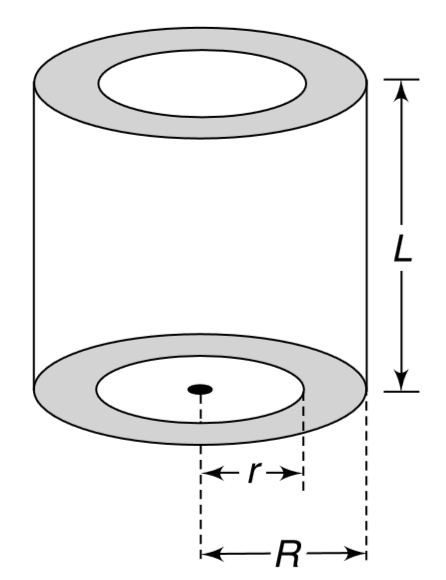# A thick cylindrical shell made of material of thermal conductivity k has inner and outer radii r and R respectively and its length is L. When the curv

90 views
in Physics
A thick cylindrical shell made of material of thermal conductivity k has inner and outer radii r and R respectively and its length is L. When the curved surface of the cylinder are lagged (i.e., given insulation cover) and one end is maintained at temperature T_(1) and the other end is maintained at T_(2)(lt T_(1)), the heat current along the length of the cylinder is H. In another experiment the two ends are lagged and the inner wall and outer wall are maintained at T_(1) and T_(2) respectively. Find the radial heat flow in this case.by (90.1k points)
Correct Answer - (2L^(2)H)/(R^(2)-r^(2)ln((R)/(r)))